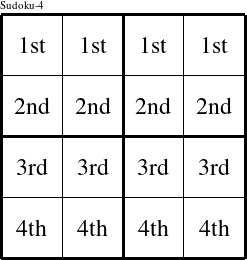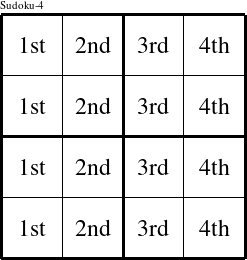#### Printable version

Detailed instructions for working a group puzzle of type 'Vern'

This is a signature puzzle named 'Vern'

The 4 elements in each group of this type of puzzle are: V, E, R, and N.

Every group in the puzzle must contain exactly this set of elements.

####In a Vern puzzle, each row is a group numbered as shown in the figure above.

####In a Vern puzzle, each column is a group numbered as shown in the figure above.

####In a Vern puzzle, each 2x2 square is a group numbered as shown in the figure above.